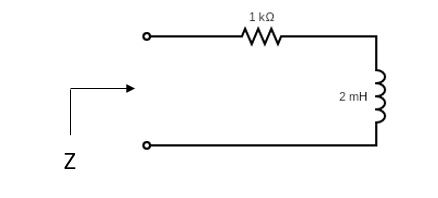## Find Impedance

Shown below is a 2 mH inductor in series with a 1 kilo-ohm resistor, and an AC voltage source operating at a frequency of 100,000 rad/s. Estimate the RL impedance for the circuit. Permissible to leave variables in the final answer.Hint
The impedance of the resistor and inductor are in series. To find the inductor impedance:
$$Z_{L}=j\omega L$$$The resistor impedance is $$Z_{R}=R=1\:k\Omega$$ The inductor impedance is $$Z_{L}=j\omega L=j(100,000)(0.002)=j200\:\Omega$$ Since the two features are in series, the RL impedance for the circuit is: $$Z=1,000\:\Omega+j200\:\Omega$$$
$$Z=1,000\:\Omega+j200\:\Omega$$\$